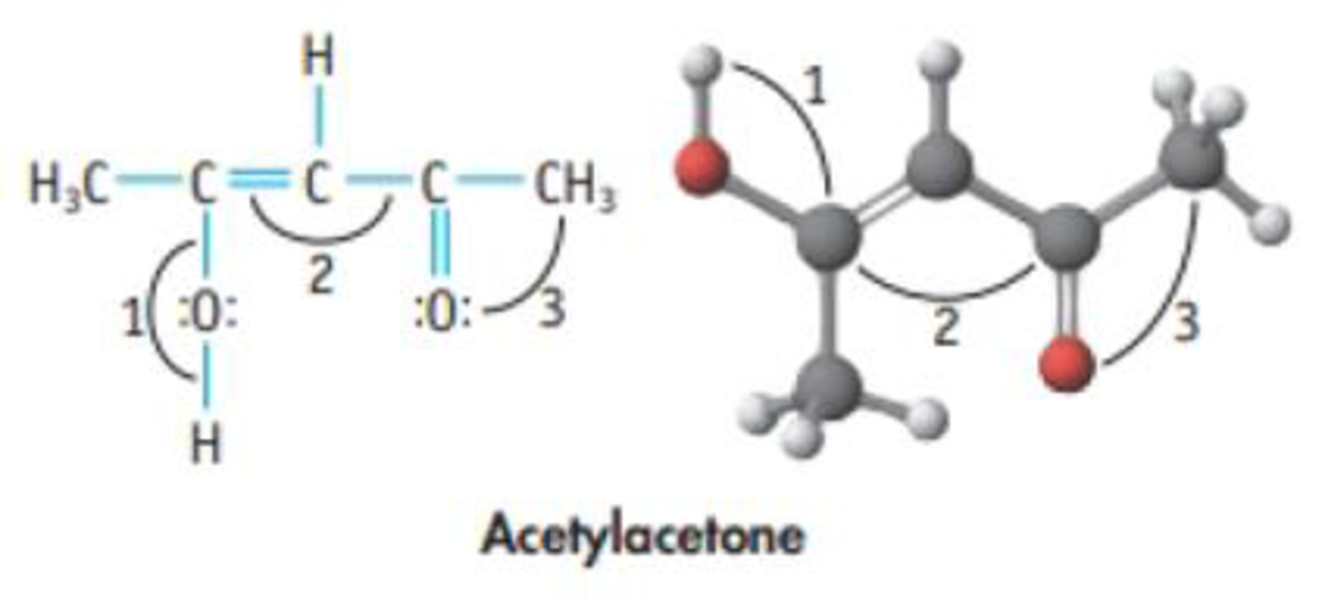Chapter 8, Problem 26PS

Chapter
Section
Textbook Problem

Acetylacetone has the structure shown here. Estimate the values of the indicated angles.Interpretation Introduction

Interpretation:

The approximate values of the angles marked 1, 2 and 3 in the given molecule should be determined.

Concept Introduction:

VSEPR Theory:

As the name itself indicates that the basis for this theory is the electron pair that is bonded electron present in either single or double bonds or lone pair electrons, present in the valence shell tends to repel each other which then tend to be in position in order to minimize the repulsions.

Bond angle: The bond angle is the angle formed between three atoms at least two bonds.

TypeofmoleculeHybridaizationGeometryBondangleAX2spLinear180°AX3,AX2Bsp2Trigonalplanar120°AX4,AX3B,AX2B2sp3Tetrahedral109.5°AX5,AX4B,AX3B2,AX2B3sp3dTrigonalbipyramidal120°,90°AX6,AX5B,AX4B2sp3d2Octahedral90°ACentralatomXAtomsbondedtoABNonbondingelectronpairsonA

Explanation

Given structure of acetylacetone molecule is,

Value of the angles marked as 1, 2, and 3 in the molecule can be determined as follows,

1 is the bond angle between COH bonds. The central oxygen atom is sp3 hybridized and so the bond angle value will be 109.5o

2 is the bond angle between C=CC bonds

Still sussing out bartleby?

Check out a sample textbook solution.

See a sample solution

The Solution to Your Study Problems

Bartleby provides explanations to thousands of textbook problems written by our experts, many with advanced degrees!

Get Started

How do eating disorders affect health?

Understanding Nutrition (MindTap Course List)

Where would you go on Earth to have a celestial pole at your zenith?

Horizons: Exploring the Universe (MindTap Course List)

Match the terms and definitions.

Human Biology (MindTap Course List)